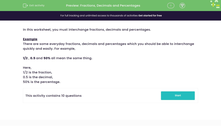# Convert Between Fractions, Decimals and Percentages

In this worksheet, students will interpret percentages as fractions and decimals, fractions as decimals and percentages, and decimals as percentages and fractions.Key stage:  KS 3

Curriculum topic:   Number

Curriculum subtopic:   Define, Interpret and Compare Percentages

Difficulty level:#### Worksheet Overview

In this activity, we will convert between fractions, decimals and percentages.

Example

There are some everyday fractions, decimals and percentages which you should be able to convert quickly and easily.

For example, 1/2 , 0.5 and 50% all mean the same thing:

1/2 is the fraction

0.5 is the decimal

50% is the percentage

For those that we haven't learned, we need to know how to convert between them.

The magic number to remember with percentages is 100!

If you are changing either a fraction or a decimal into a percentage, you multiply by 100.

If you are changing a percentage into a fraction or a decimal, you divide by 100.

It's that simple!!

Let's take a look at some examples:

3/4 as a percentage is 3/4 x 100 = 75%

0.63 as a percentage is 0.63 x 100 = 63%

60% as a fraction is 60 ÷ 100 = 60/100 which simplifies to 3/5

85% as a decimal is 85 ÷ 100 = 0.85

Have you got that!Ok, so now all we have to do is convert between fractions and decimals!

It's pretty easy to convert from a decimal to a fraction, so long as you understand place value.

0.6 means six tenths, or 6/10 which can be simplified to 3/5

0.73 means 73 hundredths or 73/100

2.09 means 2 whole numbers and 9 hundredths, so 2 9/100

This leaves us with the only tricky conversion - fractions to decimals.

Even these can be easy if the denominator is easily converted into 10 or 100.

For example, 7/20 can be easily converted into 35/100 by multiplying both the top and the bottom by 5. Once the fraction is written over 100, it is simple to make it a decimal: 35/100 is 0.35

Or 3/5 can be converted into 6/10 by multiplying both the top and the bottom number by 2. To make a decimal, 6/10 is written as 0.6

For trickier conversions, such as 3/8, we have to divide the  numerator by the denominator: 3 ÷ 8. This gives us 0.375

Right, are you ready to have a go at some questions now?

You can check back to these instructions at any point by clicking on the pink button at the side of the screen.

### What is EdPlace?

We're your National Curriculum aligned online education content provider helping each child succeed in English, maths and science from year 1 to GCSE. With an EdPlace account you’ll be able to track and measure progress, helping each child achieve their best. We build confidence and attainment by personalising each child’s learning at a level that suits them.

Get started